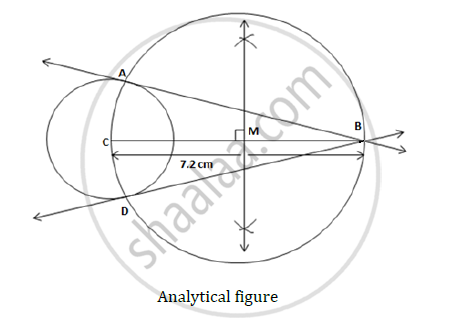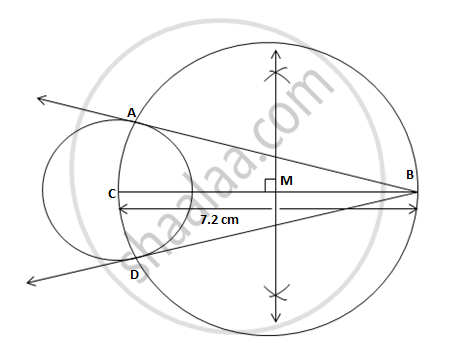# Draw tangents to the circle with center ‘C’ and radius 3.6 cm, from a point B at a distance of 7.2 cm from the center of the circle. - Geometry

Draw tangents to the circle with center ‘C’ and radius 3.6 cm, from a point B at a distance of 7.2 cm from the center of the circle.

#### Solution

Construction:-Steps of Construction:

1. Construct a circle with centre C and radius 3.6cm. Take point B such that CB =7.2cm.
2. Obtain Midpoint M of seg CB. Draw a circle with centre M and radius MB.
3. Let A and D be the points of intersection of these two circles. Draw lines BA and BD which are the required tangents.Concept: Construction of Tangents to a Circle
Is there an error in this question or solution?